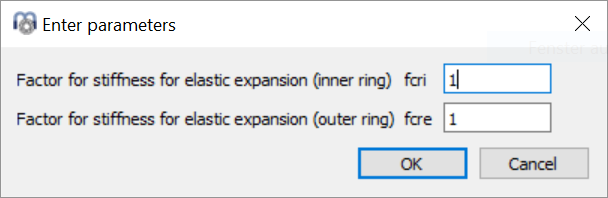﻿ Elastic ring expansion

# MESYS Calculation Software

 Elastic ring expansion

As default, only the elasticity of the contact between rolling element and race is considered. If, for example, an angular contact bearing with clearance between outer ring and housing is under axial pretension, the outer ring can expand radially. This will reduce the pretension.

In the current version, two options for consideration of elastic expansion of bearing rings are available. Both options are based on the assumption of a thick-walled ring as in the calculation of interference fits.

Either the minimal or the mean radial force in the load distribution is converted into a constant radial pressure which is then taken into account as in a calculation for interference fits. Both rings inner/outer ring and shaft/housing are considered in the calculation of the fit. This calculation approach is only valid if the variation of radial forces is small. So the axial load should normally be larger than the radial load.

The ring diameter is taken as Dpw±Dw as default, so a stiffening effect of shoulders is not taken into account. Using the-button behind the bearing type instead the use of an equivalent cross section can be activated. Then the ring diameter is defined so that the cross section is equivalent to the cross section of the bearing ring. Both options for the ring diameter can be compared with FEA results using the graphic "Radial expansion of races".

An additional factor for the ring stiffness of the inner and outer ring can be defined using the-button behind the selection box. This factor is applied to the surface used for conversion of the rolling element force to a pressure: p = F/(fcr*A).The elastic ring expansion is set to zero in case deformations from the shaft calculation are considered as the ring expansion should not be considered twice.